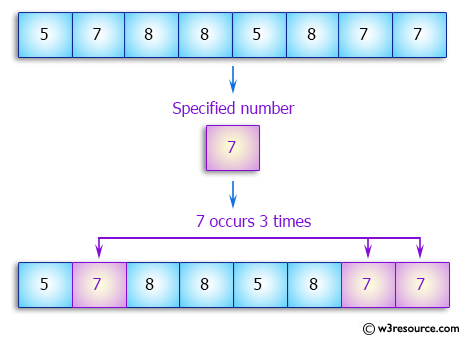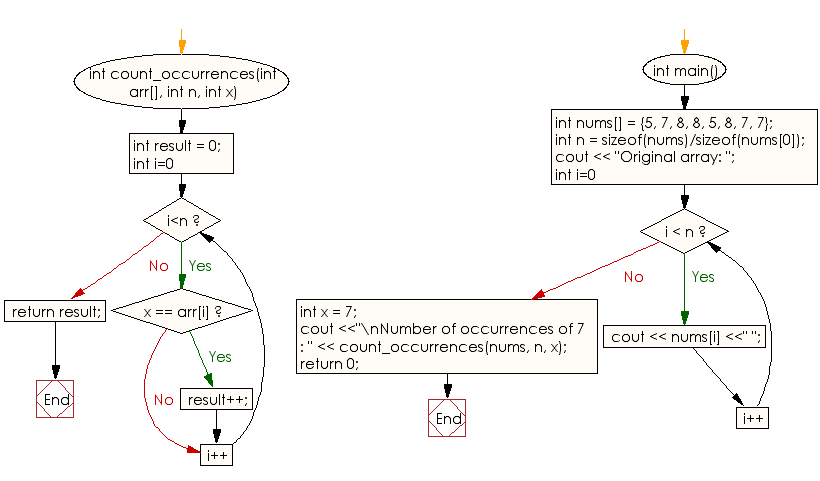﻿ C++ : Count the number of occurrences of an sorted array# C++ Exercises: Count the number of occurrences of given number in a sorted array of integers

## C++ Array: Exercise-20 with Solution

Write a C++ program to count the number of occurrences of given number in a sorted array of integers.

Pictorial Presentation:Sample Solution:

C++ Code :

``````#include <iostream>
using namespace std;

int count_occurrences(int arr[], int n, int x)
{
int result = 0;
for (int i=0; i<n; i++)
if (x == arr[i])
result++;
return result;
}

int main()
{
int nums[] = {5, 7, 8, 8, 5, 8, 7, 7};
int n = sizeof(nums)/sizeof(nums);
cout << "Original array: ";
for (int i=0; i < n; i++)
cout << nums[i] <<" ";
int x = 7;
cout <<"\nNumber of occurrences of 7 : " << count_occurrences(nums, n, x);
return 0;
}
``````

Sample Output:

```Original array: 5 7 8 8 5 8 7 7
Number of occurrences of 7 : 3
```

Flowchart:C++ Code Editor:

What is the difficulty level of this exercise?

Test your Programming skills with w3resource's quiz.

﻿